telescopeѲptics.net          ▪▪▪▪                                             CONTENTS

# 2.2. Telescope resolution

Resolution is another vital telescope function. Simply put, telescope resolution limit determines how small a detail can be resolved in the image it forms. In the absence of aberrations, what determines limit to resolution is the effect of diffraction. Being subject to eye (detector) properties, resolution varies with detail's shape, contrast, brightness and wavelength. The conventional indicator of resolving power - commonly called diffraction resolution limit - is the minimum resolvable separation of a pair of close point-object images, somewhat arbitrarily set forth by the wave theory at ~λ/D in radians for incoherent light, λ being the wavelength of light, and D the aperture diameter (expressed in arc seconds, it is 134/D for D in mm, or 4.5/D for D in inches, both for 550nm wavelength).

Resolving two point sources is inevitably dependent on telescope magnification. If the images of two point of light are to be fully resolved, they need to be separated by at least a single non-illuminated retinal photoreceptor (presumably cone, since the resolution limit of rods is significantly lower). Reaching near 100% of the diffraction limit for point-sources requires very high magnifications, but the gain in resolution is relatively small after about 25x per inch of aperture.

While there is no difference in a single point-source imaging between coherent and incoherent light with respect to the relative intensity distribution - as long as light remains near monochromatic - the resolution limit for a pair of point sources for the former varies with the phase difference between the two sources, from ~2λ/D with zero phase difference, to ~λ/D with π/2 phase difference, and about twice better than that with the phase difference equaling π (i.e. λ/2), as shown on FIG. 12 left (from Optical Imaging and Aberrations 2, Mahajan). Since, according to Van Cittert-Zernike Theorem, light arriving from stars is coherent in amateur-size telescopes, as long as it is near monochromatic, it is an interesting question how much this coherence factor, changing with the wavelength bandwidth and source OPD, influences the actual resolution limit in the field.

The point-source diffraction resolution limit for incoherent light, coherent light with λ/4 OPD between components and, perhaps, specific cases of partly coherent light, is given by ~λF, F being the ratio number of the focal length to aperture diameter (F=f/D, with f being the focal length). It is a product of angular resolution and focal length: λF=λf/D. Specifically, this is the limit to resolution for two point-object images of near-equal intensity (FIG. 12). Resolution limit can vary significantly for two point-sources of unequal intensity, as well as with other object types (FIG. 14-16).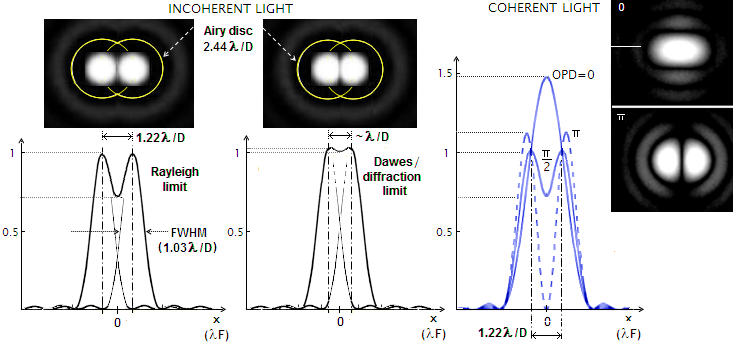FIGURE 12: LEFT: Diffraction limit to resolution of two point-object images in incoherent light is approached when the two are of near equal, optimum intensity. As the two PSF merge closer, the intensity deep between them diminishes. At the center separation of half the Airy disc diameter - 1.22λ/D  radians (138/D in arc seconds, for λ=0.55μ and the aperture diameter D in mm), known as Rayleigh limit - the deep is at nearly 3/4 of the peak intensity. Reducing separation to λ/D (113.4/D in arc seconds for D in mm, or 4.466/D for D in inches, both for λ=0.55μ) reduces the intensity deep to less than 2% below the peak. This is the conventional diffraction resolution limit for two point sources. It is just below the empirical double star resolution limit, known as Dawes' limit, given as 116/D
mm arc seconds for white stars of m~5logD-5 visual magnitude for D in mm (m~5logD+2 for D in inches), nearly identical to the Full-Width-at-Half-Maximum, or FWHM of the PSF, equaling 1.03λ/D. With further reduction in separation, the contrast deep disappears, and two spurious discs merge together. The separation at which the intensity flattens at the top is called Sparrow's limit, given by 107/D for D in mm.
RIGHT
: Resolution of two near equally bright stars in coherent light at 1.22λ/D angular separation varies with the OPD between two point sources. At zero path difference, the two patterns merge together, forming the central maxima of 1.83λF in radius and 1.47 peak intensity. At π/2 OPD the combined pattern is identical to that in incoherent light, and at OPD=π the two 1.11 maximas are somewhat more widely separated, with the intensity deep between them dropping to zero, the latter two indicating significantly better limiting resolution. Note that for given flux of x waves, individual wave amplitudes A for coherent light are first added and then squared, as (xA)
2, while squared and then added for incoherent light as xA2, in order to obtain their combined intensity. That makes the actual image intensity of coherent light for given amplitude higher by a factor of x than in incoherent light, and its change proportional to x2, not x.

Peak intensities of the two point-object images on FIG. 12 remain unchanged at the central separation of 1.22λ/D, and larger. At smaller separations (inside the Rayleigh limit), the two peak intensities start to increase, at first slowly, then rather fast, with the combined intensity doubling as the two centers merge together.

The combined intensity of the two patterns (normalized to unit peak intensity) at any point of their overlap is given by Ic=I1+I2, with I1 obtained from Eq. (c) for t1=[x-(s/2)]π/2, and I2 for t2=[x+(s/2)]π/2, with x being the point coordinate on the horizontal axis (with zero at the mid point between two PSF centroids), and s being the pattern center separation, both in units of λF

EXAMPLE: For point separation s=1.22 (Rayleigh limit), t1,2=0.305π (the sign of t is of no consequence, since it is under even number exponents), and the combined intensity at the mid point between two equal intensity patterns  Ic=0.7346, for the first four terms in the series. For one of the peak intensity points, at x1=-0.61, t1=-1.22π, t2=0 and for the other, at x2=0.61, t1=0 and t2=1.22π, with the intensity of both remaining 1.

At point separation s=1 (equaling the approximate theoretical diffraction limit of resolution for point-sources, given by λ/D in radians), the combined intensity at the mid point (x=0, t1,2=π/4) is 1.042. The two peak intensity points shift closer, away from the original centroids at xc=0.5, to  x1=-0.32 (t1=-0.41π, t2=0.09π), and x2=0.32, (t1=-0.09π, t2=0.41π), with their intensity only slightly higher, at 1.060.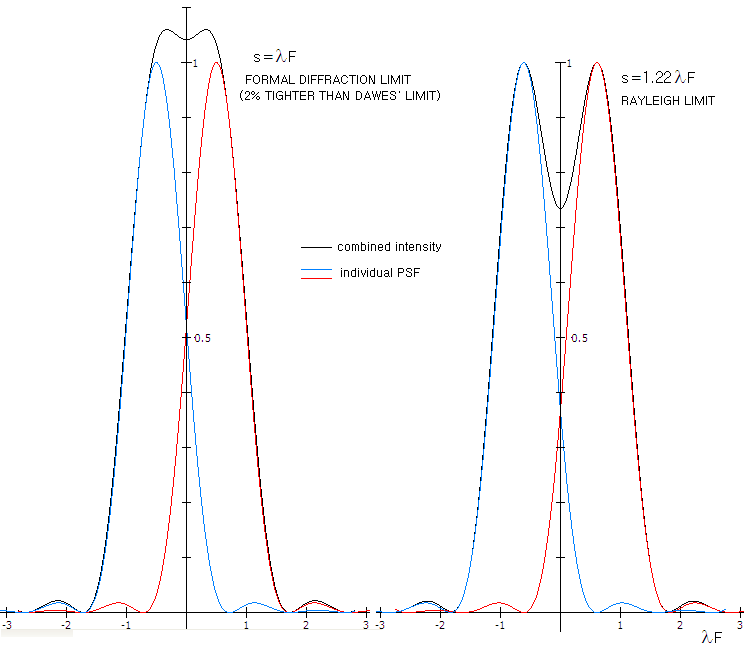At s=1.02, which is formally given for the empirical Dawes' limit, the combined intensity at the mid point (x=0, t1,2=1.02π/4) is 1.013, with the peak intensity points also shifting closer from their respective original centroids to  x1=-0.4 (t1=-0.455π, t2=0.055π), and x2=0.4, (t1=-0.055π, t2=0.455π), reaching 1.045 peak intensity. This is only slightly more than 3% difference in contrast, and considered below detection threshold of the human eye in field conditions. Formally acceptable limit should satisfy ~5% minimum contrast, which would require nearly 2% increase in separation, to s~1.04 (Suiter may be giving similar hint on p287, where he states that the separation in Dawes' criterion is "little less than 85%" of the Rayleigh limit; the exact number, as given by Sidgwick, is 83.7%). In any instance, the difference is negligible: satisfying the 5% minimum contrast differential requirement with Dawes' limit would take as little as reducing 550nm wavelength by ~2%.

The separation at which the combined PSF flattens at the top occurs at the center separation 107/D in arc seconds, for D in mm (4.2/D for D in inches). It is so called Sparrow's limit, allowing detection  of close doubles based on visual elongation of the bright central spot of diffraction pattern. For closer separations, peak intensity of the combined pattern forms at the mid point between two Gaussian point-object images.

The PSF plots above are for the nominal (normalized) intensity. While it is rather common way of illustrating point-source resolution, the human eye response to light intensity is mainly logarithmic, hence better illustrated with logarithmic PSF. For instance, the intensity gap between central peak and second maxima in aberration-free aperture is 57 to 1, respectively; the eye, however, sees the peak as less than twice brighter (this applies when both are well within eye's detection threshold; as the fainter 1st bright ring nears detection threshold and falls bellow it, the perceived intensity differential dramatically increases). Graph below (FIG. 13) shows logarithmic (log10) PSF for polychromatic light (in the range that is 1/10 of the mean wavelength, inset H), closer to the PSF of an actual star than monochromatic PSF.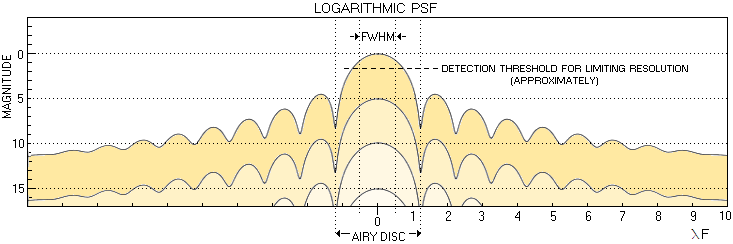FIGURE 13
: Logarithmic PSF of aberration-free aperture on the (stellar) magnitude scale shows intensity distribution within stellar image more closely to that actually perceived by human eye (i.e. the apparent intensity scales inversely with magnitude). Going from zero magnitude star to magnitude 15, there is no indication that visual size of the central maxima differs considerably between bright vs. average and moderately faint stars (this neglects possible - and probable - secondary physiological effects on the retina, particularly with very bright sources). Only as the outskirts of the central maxima begin to fall below detection threshold, its visible size diminishes. For the maximum theoretical resolution of two point-sources, set at λ/D in radians (206,265λ/D in arc seconds), visible central disc cannot be significantly larger than λ/D angularly (illustrated for the zero magnitude star, for convenience). Moderately larger disc still should allow clear resolution, due to the intensity low forming between two star images, with the discs likely appearing less than perfectly round. The graph above implies that it would take place at the detection threshold approximately two magnitudes below the peak intensity. This is not far from the reported basis in establishing empirical resolution limit by Rev. William Rutter Dawes: near equally bright pairs about three magnitude brighter than the faintest star detectable with the aperture tested (Sky Catalogue 2000.0, Hirshfeld/Sinnott, p.xi). According to it, limiting resolution is possible only in the absence of visible ring structure (typical aberration level, or average central obstruction, brighten the 1st bright ring less than a magnitude - as illustrated on FIG. 95 - which amounts to ~2mm height differential on the above graph).

As mentioned, this limit applies to near-equally bright, contrasty point-object images at the optimum intensity level. Resolution limit for star pairs of unequal brightness, or those significantly above or below the optimum intensity level is lower. For other image forms, resolution limit also can and does deviate significantly, both, above and below the conventional limit. One example is a dark line on light background, whose diffraction image is defined with the images of the two bright edges enclosing it. These images are defined with the Edge Spread Function (ESF), whose configuration differs significantly from the PSF (FIG. 14). With its intensity drop within the main sequence being, on the other hand, quite similar to that of the PSF, resolution of this kind of detail is more likely to be limited by detector sensitivity, than by diffraction (in the sense that the intensity differential for the mid point between Gaussian images of the edges vs. intensity peaks, forms a non-zero contrast differential for any finite edge separation).FIGURE 14: Limit to diffraction resolution vary significantly with the object/detail form. Image of a dark line on bright background is a conjunction of diffraction images of the two bright edges, described by Edge Spread Function (ESF). As the illustration shows, the gap between two intensity profiles at λ/D separation is much larger for the ESF than PSF (which is nearly identical to the Line Spread Function, determining the limiting MTF resolution). It implicates limiting resolution considerably better than λ/D, which agrees with practical observations (Cassini division, Moon rilles, etc.). Gradual intensity falloff at the top of the intensity curve around the edges can produce very subtle low-contrast features, even if the separation itself remains invisible.

Diffraction image of a point source on the surface of most extended objects could be detected only if separated from the rest of surface, not because it is small and relatively faint, but because it is typically of much lower intensity than that of the surface. For instance, Jupiter's total average brightness is as if it's having a ~6th magnitude star in each square arc second of its surface. Is 1 square arc second emitting area as a valid point source? It could be, but it really depends on the aperture size. Diffraction calculation (Imaging and aberrations 2, Mahajan) shows that the light-emitting incoherent disc - or a hole - smaller than ~1/4 of the Airy disc, produces PSF no appreciably different than that of a perfect point source (FIG. 14). With the angular Airy disc diameter given by 2.44λ/D in radians (multiplied by 206,265 for arc seconds), that sets the maximum disc (hole) diameter that qualifies as a point-source at ~0.6λ/D, or smaller, in radians, ~125,000λ/D, or smaller, in arc seconds (the corresponding linear size is directly determined by its distance, as a product of the distance and its angular size in radians).

Consequently, diffraction image of an extended surface can be evaluated as a product of surface dots not larger than 1/4 of the Airy disc diameter (further division of this effective point-source at given surface luminance merely diminishes the actual PSF maxima of such surface unit, but its spatial characteristics do not change appreciably vs. that for 1/4 Airy disc dot, nor the PSF volume integrated over 1/4 Airy disc dot area does differ appreciably from one produced by such dot). In terms of square arc seconds, the area corresponding to 125,000λ/D diameter dot is for the square side smaller by a factor of π/4, thus given by 99,000λ/D. For λ=0.00055mm (photopic peak), that would give 0.54 square arc second (i.e. square with a 0.54 arc second side) for 100mm aperture, 0.27 arc seconds for 200mm, and so on.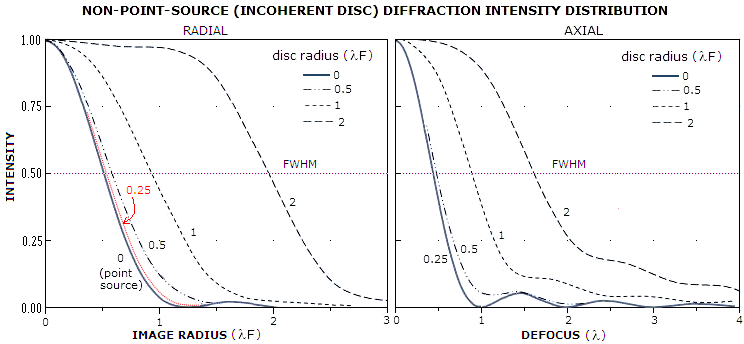FIGURE 15: An object does not have to be strictly a point-source in order to produce point-source PSF, but if its angular dimensions exceed certain level, its central diffraction maxima widens, and it transforms into an image of extended object. LEFT: Change in the radial intensity distribution as emitting area increases from zero (point-source) to a disc 2λF in radius. At the disc radius equaling λF/4, or 1/5 of the Airy disc radius, resulting PSF is only slightly wider than that of a point-source, hence a circular emitting area of that size, or smaller, can be considered a point-source with respect to its diffraction image. RIGHT: Change in the central intensity with the increase in axial defocus. The larger disc radius, the less sensitive to defocus is the central intensity of its image. While it drops to zero already at 1 wave defocus for a disc (hole) radius equaling λF/4, it remains above zero beyond 4 wave defocus already at a disc radius equaling λF, slightly smaller than the Airy disc's. Note that central intensities on both graphs are all normalized to 1, but the actual peak intensity vary with the disc size. With constant disc's surface luminance, the actual diffraction peaks for 0.25, 0.5, 1 and 2 radii, normalized to the highest, would relate as 0.15, 0.88, 0.97 and 1, respectively.

Unlike point-source diffraction image, where there is no appreciable difference in the shape of normalized PSF for coherent and incoherent light, an extended object image in coherent light develops isolated peaks over its central maxima, the strongest being at its edge. This results in the effect called "edge ringing", making integrity of the image inferior to that in incoherent light.

Surface of an extended object can be decomposed on point-sources, that overlap and grow into a larger diffraction image of it. Any distinctive area on such surface also can be decomposed on its effective point-sources. Whether such an area - a surface detail - will be visible in the telescope image depends on the multiple factors: its size, brightness and contrast and, if colors are present, hue specificity and saturation.

Of course, optical aberrations can also have significant effect on the intensity distribution, image vs. object, scattering energy and lowering contrast/resolution. While aberrations here do cause the same general effect, the specifics are different than those for point-source (FIG. 16).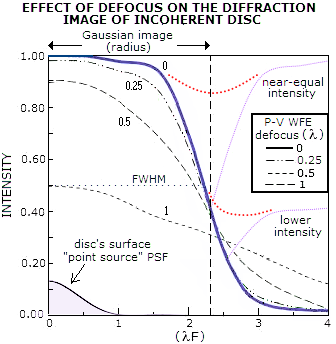FIGURE 16: Radial intensity distribution within diffraction image of incoherent disc, with the radius 2.3 times the Airy disc radius with zero defocus (solid black) and specified amounts of the aberration. As much as 1/4 wave P-V of defocus has negligible effect on both, central intensity, and energy lost to the central maxima, and as much as 1/2 wave only lowers this maxima's central intensity to 0.91. One wave of defocus, which brings central intensity of the PSF to zero, is still just below 0.5 here. However, the numerical value of central intensity here does not have the same implications as with the PSF. While in the latter it closely approximates the relative energy preserved in the maxima - hence directly implying the relative energy loss - here it is generally optimistic in that respect. The reason is the different manner with which the aberration affects the shape of central maxima: since its energy is proportional to its volume, the reshaped aberrated volume which, unlike PSF maxima, loses relatively more energy off the sides than off the top of the aberrated central maxima, causes significant disparity between the relative nominal drop in central maxima and the relative loss of energy. In general, the latter is significantly higher. So, for instance, while the drop in central maxima for 1/4 and 1/2 wave P-V of defocus is 2% and 9%, the corresponding loss of energy is - very roughly - closer to 10% and 30%, respectively. At the same time, change in the relative size of FWHM for these error levels, similarly to PSF, remains insignificant.

If the effect of aberrations on the diffraction image of an extended object is so much smaller, how do aberrations in this range, quite common in telescopes, inflict noticeable loss to the contrast of extended detail? Well, they don't; not at this detail size level. With the Gaussian image radius of 2.3λF, this disc is nearly 4.5 times wider than the MTF cutoff frequency (1.03λF), which puts the corresponding normalized MTF frequency at 0.22. Thus it is in the low-frequency domain where the contrast drop caused by aberrations is generally lower (FIG. 17).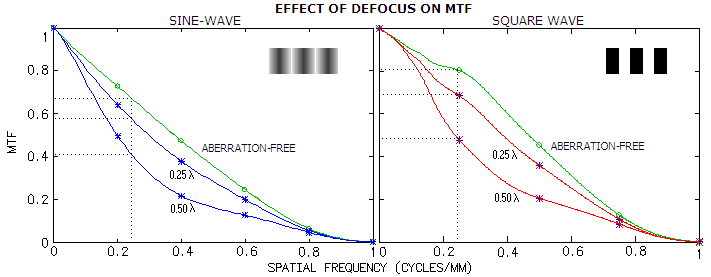FIGURE 17: Polychromatic (photopic) MTF plots at left showing effect of defocus on contrast transfer and, for comparison, their effect on CTF (right). The sine-wave (standard) MTF has generally lower contrast transfer than square-wave CTF, with defocus in the former lowering contrast vs. aberration-free image at 0.22 frequency 14% at 1/4 wave P-V, and 39% at 1/2 wave. That compares to 19% and 56% contrast loss, respectively, averaged over full range of frequencies. With the square-wave CTF, the corresponding contrast loss is 14% and 40%, respectively.

Both MTF and CTF give contrast loss at this detail size greater than the rough estimate of the energy/contrast loss based on radial energy distribution. The difference is relatively modest at 1/4 wave of defocus, 14% vs. ~10%, and more ambivalent at 1/2 wave: 56% and 40% vs. ~30% for the MTF and CTF, respectively. But it is to expect, since neither is directly comparable in the form to a coherent disc (at 1/2 wave defocus error, the contrast transfer difference between the two is even slightly greater than between the CTF and the disc).

And neither of the two MTFs nor, for that matter, incoherent disc on dark background, are a detail form similar to, say, typical planetary detail. Such detail is imbedded into surrounding of adjacent details of similar intensity. The level of its detection depends as much - if not more - on color distinction, as on intensity differential (contrast). The color factor is entirely neglected by MTF. If two objects of the same intensity are placed in contact with each other, their image will show a continuous, single surface, simply because there is no discontinuity in wave emission. But if these surfaces emit at different principal wavelengths, the eye will create distinction by assigning to them different colors. In other words, color produces contrast-like quality, which can enhance detection/resolution for any level of image-inherent contrast, including zero.

If we, however, assume that such extended details are not seamlessly bonded to their surroundings, and/or vary in their relative intensities - the more likely scenario - then there is wave emission discontinuity between them, and their diffraction images, at least in first approximation, superimpose forming the complex final image. Between two very close details of similar intensity - as illustrated on FIG. 10C top right,  the combined energy will likely fill out most of the gap between their respective individual images, leaving only a narrow, very low-contrast transition area, unlikely to be detected. Detection of such details would depend entirely on their color distinction; the lower it is, the sooner it will be affected by aberration-caused spread of energy, but the degree to which it will be affected also critically depends on detail's angular size.

If detail's relative intensity is significantly different, contrast also becomes significant factor (FIG. 10C, bottom right). Such details are more typical of the Moon surface. Due to their relatively high contrast level, they will be less affected by the aberrated energy spilling over. Again, their angular size is the major determinant of the effect of any given aberration level on their detection.

This, obviously, only scratches the surface of the relation between image quality of extended details and aberrations. But this very basic concept does shed more light on this fairly obscured subject. In general, larger aperture will resolve more, because its effective point-source (which can be also seen as image pixel) is, as mentioned, inversely proportional to the aperture size. Also, it will have better color saturation. The brightness factor is somewhat ambivalent, since it can be both, beneficial and detrimental. It is generally beneficial in detection of point-sources and alike, as well as faint objects of all types. It can be disadvantageous for resolution of bright point-like and extended objects' details. However, since telescope light transmission can be easily lowered at any given aperture, that detriment is of rather formal nature.

Generally, the size of smallest detectable detail on the surface of an extended object is roughly proportional to the telescope's nominal (point-object) diffraction resolution limit and light gathering power, but it is also significantly lower, varying with the detail type and surrounding. For the typical bright low-contrast details (major planets), and dim low-contrast details (most nebulas and galaxies), the MTF analysis by Rutten and Venrooij (Telescope Optics, p215) indicates the MTF resolution limit lower approximately by a factor of ~2 and ~7, respectively, than for bright, contrasty pattern (which is practically identical to the telescope's nominal stellar resolution limit).

Formal premises and experimental results on the subject of telescope resolution are covered in detail in Amateur Astronomer's Handbook, J.B. Sidgwick (p37-51). Naturally, resolution in general will deteriorate with introduction of wavefront aberrations.